# Machine Learning Instance Based Learning Adapted from various

• Slides: 15Machine Learning Instance Based Learning (Adapted from various sources)Introduction • Instance-based learning is often termed lazy learning, as there is typically no “transformation” of training instances into more general “statements” • Instead, the presented training data is simply stored and, when a new query instance is encountered, a set of similar, related instances is retrieved from memory and used to classify the new query instance • Hence, instance-based learners never form an explicit general hypothesis regarding the target function. They simply compute the classification of each new query instance as neededk-NN Approach • The simplest, most used instance-based learning algorithm is the k-NN algorithm • k-NN assumes that all instances are points in some n-dimensional space and defines neighbors in terms of distance (usually Euclidean in R-space) • k is the number of neighbors consideredGraphic Depiction Properties: 1) All possible points within a sample's Voronoi cell are the nearest neighboring points for that sample 2) For any sample, the nearest sample is determined by the closest Voronoi cell edge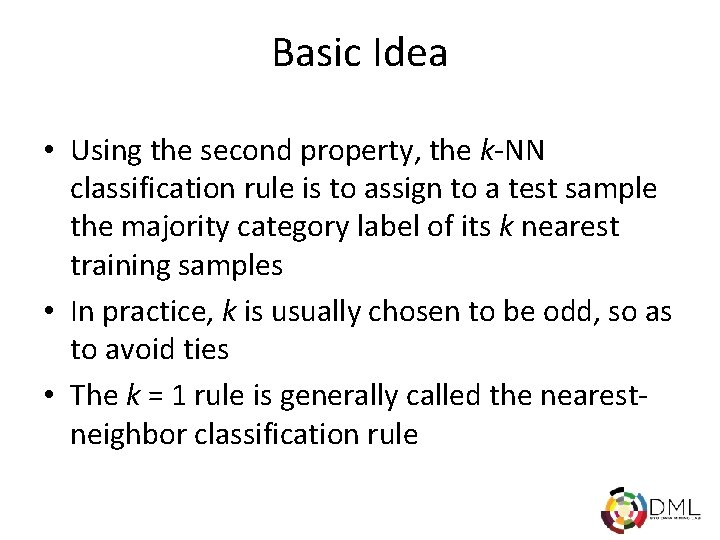Basic Idea • Using the second property, the k-NN classification rule is to assign to a test sample the majority category label of its k nearest training samples • In practice, k is usually chosen to be odd, so as to avoid ties • The k = 1 rule is generally called the nearestneighbor classification rulek-NN Algorithm • For each training instance t=(x, f(x)) – Add t to the set Tr_instances • Given a query instance q to be classified – Let x 1, …, xk be the k training instances in Tr_instances nearest to q – Return • Where V is the finite set of target class values, and δ(a, b)=1 if a=b, and 0 otherwise (Kronecker function) • Intuitively, the k-NN algorithm assigns to each new query instance the majority class among its k nearest neighborsSimple Illustration q is + under 1 -NN, but – under 5 -NN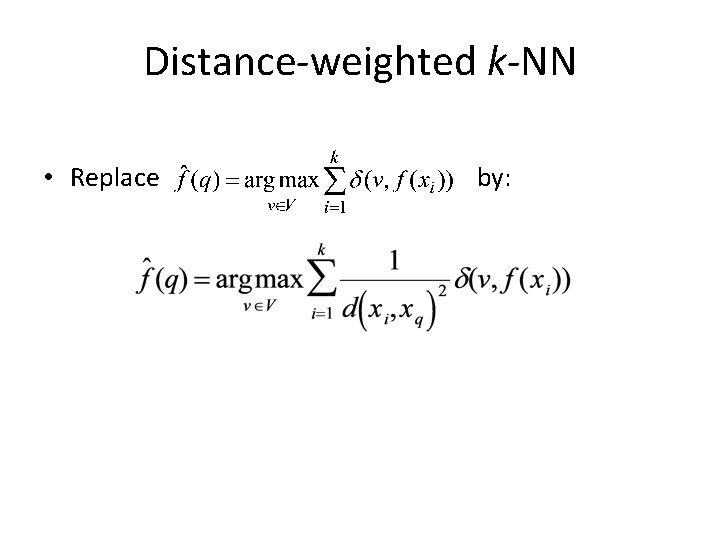Distance-weighted k-NN • Replace by: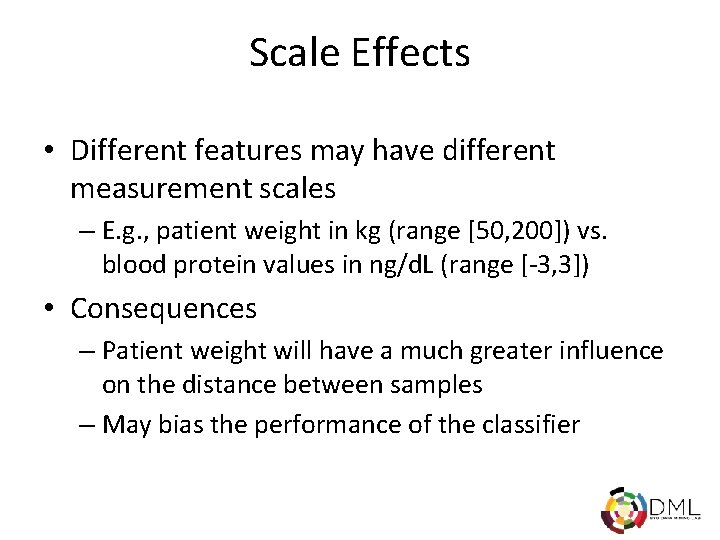Scale Effects • Different features may have different measurement scales – E. g. , patient weight in kg (range [50, 200]) vs. blood protein values in ng/d. L (range [-3, 3]) • Consequences – Patient weight will have a much greater influence on the distance between samples – May bias the performance of the classifier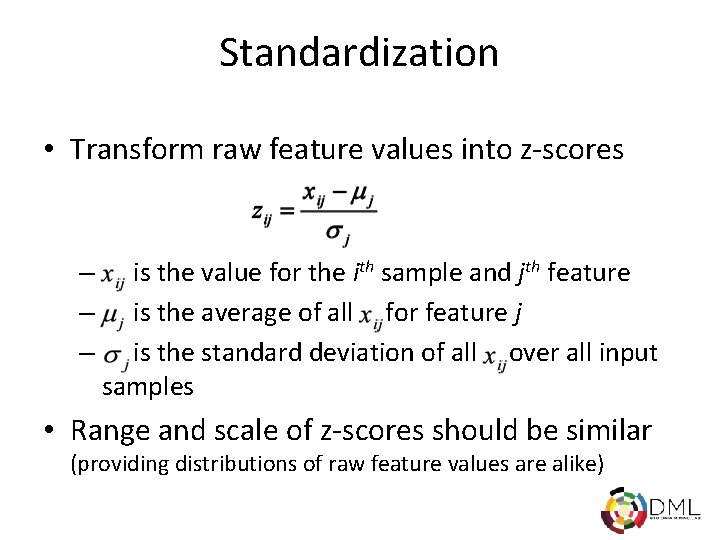Standardization • Transform raw feature values into z-scores – – – is the value for the ith sample and jth feature is the average of all for feature j is the standard deviation of all over all input samples • Range and scale of z-scores should be similar (providing distributions of raw feature values are alike)Distance MetricsIssues with Distance Metrics • Most distance measures were designed for linear/real-valued attributes • Two important questions in the context of machine learning: – How best to handle nominal attributes – What to do when attribute types are mixed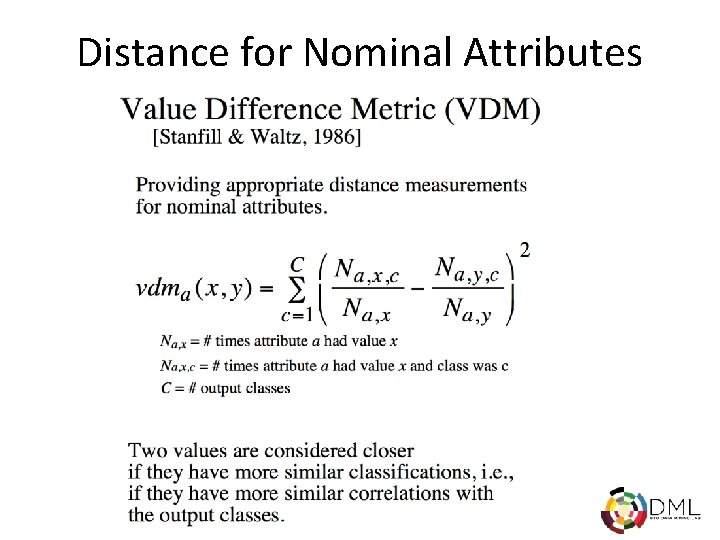Distance for Nominal AttributesDistance for Heterogeneous Data Wilson, D. R. and Martinez, T. R. , Improved Heterogeneous Distance Functions, Journal of Artificial Intelligence Research, vol. 6, no. 1, pp. 1 -34, 1997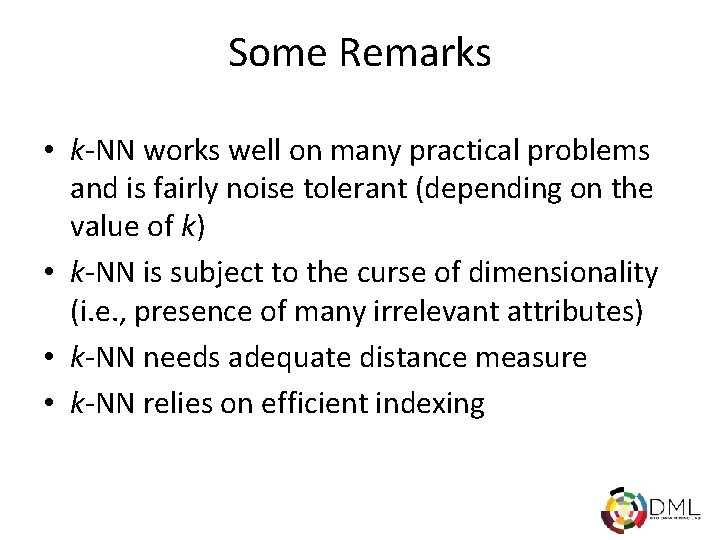Some Remarks • k-NN works well on many practical problems and is fairly noise tolerant (depending on the value of k) • k-NN is subject to the curse of dimensionality (i. e. , presence of many irrelevant attributes) • k-NN needs adequate distance measure • k-NN relies on efficient indexing